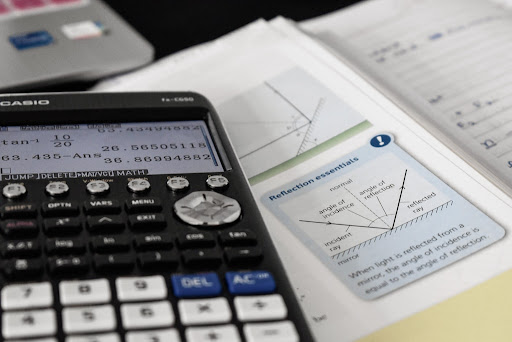# What is a slope in math and how to calculate it?

0
175The Slope calculator can help you to determine the slope of a line that passes through 2 points.

This post will briefly explain a slope in math, calculate a slope, and define a slope in math.

In mathematics, the slope of a line is a measurement of the line’s steepness and direction. Without using a compass, determining the slope of lines in a coordinate plane can aid in anticipating whether they are parallel, perpendicular, or none. The general formula for a line’s slope is y = mx + b.

Determine the coordinates of two points on the line. Next, calculate the difference between these two locations’ y-coordinates (rise). Next, calculate the x-coordinate difference between these two places (run). Finally, divide the y-coordinate difference by the x-coordinate difference (rise/run or slope).

## How to calculate slope with two points?

Any two different points on a line may be used to compute the slope of the line. The slope of a line formula determines the proportion of “vertical change” to “horizontal change” between two points on a line. We will learn about the slope-finding method and its applications in this post.

We will now explain the easiest way to find a slope. Different approaches may be used to determine the slope of a line. The first approach for determining the slope value is to use the equation $m=\frac{y_2-y_1)}{x_2-x_1}$, where m is the line’s slope. In addition, the change in x is referred to as a run, while the change in y is referred to as a rise or fall. As a result, a slope may alternatively be defined as m = rise/run.

Check out our online slope calculator – https://calconcalculator.com/math/slope-calculator/

## How to calculate the angle of a slope?

In this section, we will explain how we can calculate the slope angle. So, the angle produced by the run (remember, it’s an idealized flat surface that ignores elevation change) and the angular departure of your climb from that idealized flat surface is called the angle of slope. To do so, divide the rise by the run, then get the inverse tangent of the result.

## What is the formula to find a slope?

$m=\frac{y_2-y_1)}{x_2-x_1}$

It’s worth noting that a line’s slope may be readily determined by hand using tiny, whole integer coordinates. The formula becomes more useful as the coordinates get bigger or have decimal values.

Because horizontal lines have the same y-coordinates, they all have a gradient of zero. In the numerator of the slope formula, this will result in a zero. In contrast, because the x-coordinates are always the same, a vertical line will have an indeterminate slope. When employing the formula, this will produce a division by zero mistakes.

## How to calculate slope percentage?

The elevation change is divided by the amount of horizontal distance covered (often referred to as “the climb divided by the run”). The result is multiplied by 100 to get the percent of slope. The “run” implies you’re on a perfectly level surface and doesn’t take into account the actual distance covered after elevation change is taken into account.

Assume your ascent acquires 1,000 feet in height (the increase) and a horizontal distance of 2,000 feet as indicated on the map (the run).

0.5 is equal to 1,000 divided by 2,000.

To get the percent of a slope, multiply 0.5 by 100, which is 50%